Analysis WebNotes
arrow_back arrow_forward

Class Contents

Closed Sets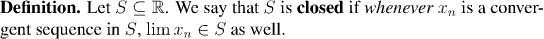Example: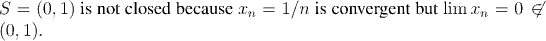Example:
Any closed interval [a,b] is closed.

Example: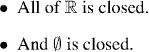Example:
Any finite set is closed.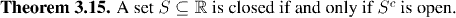Example: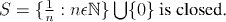Example:

We can construct a very interesting closed set called the "Cantor middle-1/3 set" by starting with the closed unit interval [0, 1] and repeatedly removing the middle 1/3 from each of the intervals left over from the previous step. The result of the process is the set remaining after all the open intervals in this (infinite) process have been removed.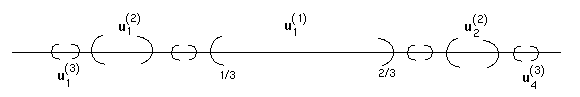Remark:
Remember that the union of a collection of open sets is also open. The corresponding result for closed sets is: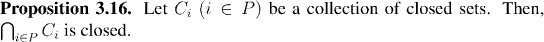I want to show you two ways of proving this. One, directly, and the other, by deriving it from what we already know about open sets.

To see another, quicker way of proving the last Proposition, we use the following factoid:

Factoid: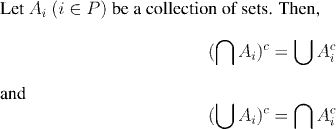Thus, we can use Proposition 3.15 by to prove Proposition 3.16 as folllows: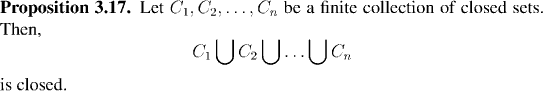Example:Example: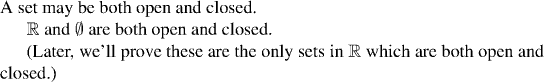Example: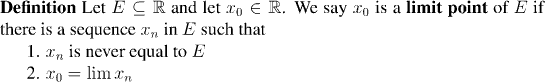Example: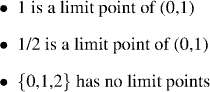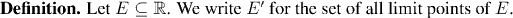Example: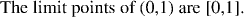The proof is in the homework.

Remark: